Open in App
Not now

# Non-Access Modifiers in Java

• Last Updated : 01 Dec, 2021

Modifiers are specific keywords present in Java using that we can make changes to the characteristics of a variable, method, or class and limit its scope. Java programming language has a rich set of Modifiers.

Modifiers in Java are divided into two types – Access Modifiers and Non-Access modifiers

Access Modifiers in Java help restrict the scope of a variable, method, class, or constructor. Public, Private, Protected, and Default these four access modifiers are present in Java.

### Non-Access modifiers

Non-access modifiers provide information about the characteristics of a class, method, or variable to the JVM. Seven types of Non-Access modifiers are present in Java. They are –

1. static
2. final
3. abstract
4. synchronized
5. volatile
6. transient
7. native

### 1. static

The static keyword means that the entity to which it is applied is available outside any particular instance of the class. That means the static methods or the attributes are a part of the class and not an object. The memory is allocated to such an attribute or method at the time of class loading. The use of a static modifier makes the program more efficient by saving memory. A static field exists across all the class instances, and without creating an object of the class, they can be called.

Example 1:

## Java

 `import` `java.io.*;`` ` `// static variable``class` `static_gfg {``    ``static` `String s = ``"GeeksforGeeks"``; ``}``class` `GFG {``    ``public` `static` `void` `main(String[] args)``    ``{``        ``// No object required``        ``System.out.println(``            ``static_gfg.s); ``    ``}``}`

Output

`GeeksforGeeks`

In this above code sample, we have declared the String as static, part of the static_gfg class. Generally, to access the string, we first need to create the object of the static_gfg class, but as we have declared it as static, we do not need to create an object of static_gfg class to access the string. We can use className.variableName for accessing it.

Example 2:

## Java

 `import` `java.io.*;`` ` `class` `static_gfg {`` ` `    ``// static variable``    ``static` `int` `count = ``0``; ``    ``void` `myMethod()``    ``{``        ``count++;``        ``System.out.println(count);``    ``}``}``class` `GFG {``    ``public` `static` `void` `main(String[] args)``    ``{``        ``// first object creation``        ``static_gfg obj1 = ``new` `static_gfg(); ``           ` `        ``// method calling of first object``        ``obj1.myMethod(); ``           ` `        ``// second object creation``        ``static_gfg obj2``            ``= ``new` `static_gfg(); ``       ` `        ``// method calling of second object``        ``obj2.myMethod(); ``    ``}``}`

Output

```1
2```

In the above code, the count variable is static, so it is not tied to a specific instance of the class. So, while obj1.myMethod() is called it increases the value of count by 1 and then obj2.myMethod() again increases it . If it was not a static one, then we will get output as 1 in both cases, but as it is a static variable so that count variable will be increased twice, and we will get 2 as an output the second time.

### 2. final

The final keyword indicates that the specific class cannot be extended or a method cannot be overridden. Let’s understand that with an example –

Example 1:

## Java

 `import` `java.io.*;`` ` `class` `final_gfg { ``    ``String s1 = ``"geek1"``;``}``class` `extended_gfg ``extends` `final_gfg { ``                         ` `    ``String s2 = ``"geek2"``;``}``class` `GFG {``    ``public` `static` `void` `main(String[] args)``    ``{``      ``// creating object``      ``extended_gfg obj = ``new` `extended_gfg(); ``       ` `      ``System.out.println(obj.s1);``      ``System.out.println(obj.s2);``    ``}``}`

Output

```geek1
geek2```

In this above code, the final_gfg class is extended by the extended_gfg class, and the code is working fine and producing output.

But after using the final keyword with the final_gfg class. The code will produce an error. Below is the implementation for the same –

## Java

 `import` `java.io.*;`` ` `// This class is final``final` `class` `final_gfg { ``    ``String s1 = ``"geek1"``;``}``// We are trying to inherit a final``class` `extended_gfg ``extends` `final_gfg { ``                         ` `    ``String s2 = ``"geek2"``;``}``class` `GFG {``    ``public` `static` `void` `main(String[] args)``    ``{``        ``// creating object``        ``extended_gfg obj``            ``= ``new` `extended_gfg();``       ` `          ``System.out.println(obj.s1);``          ``System.out.println(obj.s2);``    ``}``}`

Error :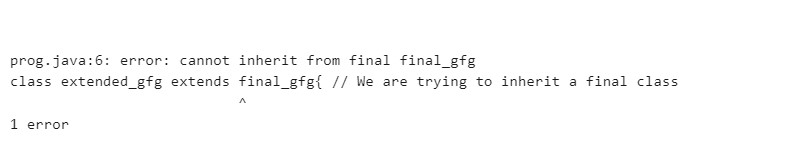Screenshot of Error

Here we are getting errors in the compilation as we are trying to extend the final_gfg class, which is declared as final. If a class is declared as final, then we cannot extend it or inherit from that class.

Example 2:

## Java

 `import` `java.io.*;`` ` `class` `final_gfg{``     ``void` `myMethod(){``        ``System.out.println(``"GeeksforGeeks"``);``    ``}``}``class` `override_final_gfg ``extends` `final_gfg{``    ``void` `myMethod(){ ``        ``System.out.println(``"Overrides GeeksforGeeks"``);``    ``}``}`` ` `class` `GFG{``    ``public` `static` `void` `main(String[] args) {``        ``override_final_gfg obj=``new` `override_final_gfg();``        ``obj.myMethod();``    ``}``}`

Output

`Overrides GeeksforGeeks`

In the above code, we are overriding myMethod(), and the code is working fine.

Now we are going to declare the myMethod() in superclass as final. Below is the implementation for the same –

## Java

 `import` `java.io.*;`` ` `class` `final_gfg{``    ``final` `void` `myMethod(){``        ``System.out.println(``"GeeksforGeeks"``);``    ``}``}``class` `override_final_gfg ``extends` `final_gfg{``    ``// trying to override the method available on final_gfg class``    ``void` `myMethod(){ ``        ``System.out.println(``"Overrides GeeksforGeeks"``);``    ``}``}``class` `GFG{``    ``public` `static` `void` `main(String[] args) {``        ``override_final_gfg obj=``new` `override_final_gfg();``        ``obj.myMethod();``    ``}``}`

Error: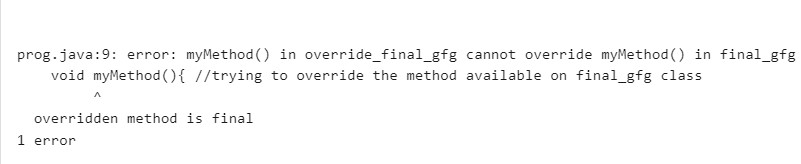Screenshot of Error

The above code is producing an error because here, we are trying to override a method that is declared as final. myMethod() in the final_gfg class is declared as final, and we are trying to override that from the override_final_gfg class. A final method cannot be overridden; thus, the code snippet is producing an error here.

### 3. abstract

abstract keyword is used to declare a class as partially implemented means an object cannot be created directly from that class. Any subclass needs to be either implement all the methods of the abstract class, or it should also need to be an abstract class. The abstract keyword cannot be used with static, final, or private keywords because they prevent overriding, and we need to override methods in the case of an abstract class.

## Java

 `// abstract class``abstract` `class` `abstract_gfg{ ``        ``abstract` `void` `myMethod();``}`` ` `//extending abstract class``class` `MyClass ``extends` `abstract_gfg{ ``   ` `    ``// overriding abstract method otherwise``    ``// code will produce error``    ``void` `myMethod(){ ``        ``System.out.println(``"GeeksforGeeks"``);``    ``}``}``class` `GFG{``    ``public` `static` `void` `main(String[] args) {``        ``MyClass obj=``new` `MyClass();``        ``obj.myMethod(); ``    ``}``}`

Output

`GeeksforGeeks`

In the above code, abstract_gfg is an abstract class, and myMethod() is an abstract method. So, we first need to extend the abstract_gfg class that we have done here using MyClass. After extending, we also need to override the abstract method otherwise, the code will produce errors.

### 4. synchronized

synchronized keyword prevents a block of code from executing by multiple threads at once. It is very important for some critical operations. Let us understand by an example –

## Java

 `import` `java.io.*;`` ` `class` `Counter{``    ``int` `count;``    ``void` `increment(){``        ``count++;``    ``}``}``class` `GFG{``    ``public` `static` `void` `main(String[] args) ``throws` `InterruptedException {``        ``Counter c=``new` `Counter();``       ` `        ``// Thread 1``        ``Thread t1=``new` `Thread(``new` `Runnable() {``            ``@Override``            ``public` `void` `run() {``                ``for``(``int` `i=``1``;i<=``10000``;i++){``                    ``c.increment();``                ``}``            ``}``        ``});``       ` `        ``// Thread 2``        ``Thread t2=``new` `Thread(``new` `Runnable() {``            ``@Override``            ``public` `void` `run() {``                ``for``(``int` `i=``1``;i<=``10000``;i++){``                    ``c.increment();``                ``}``            ``}``        ``});`` ` `        ``t1.start();``        ``t2.start();``        ``t1.join();``        ``t2.join();`` ` `        ``System.out.println(c.count);``    ``}``}`

Output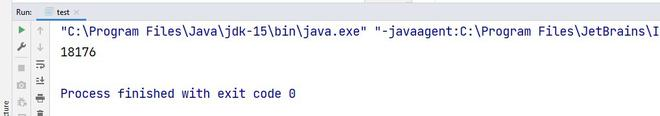The above code should be an output value of 20000 as two threads increment it 10000 times each, and the main is waiting for Thread1, Thread2 to finish. Sometimes it may not be true. Depending upon the system, it may not give 20000 as output.  As both threads are accessing the value of count, it may happen that Thread1 fetches the value of count, and before it could increment it, the Thread2 reads the value and increments that. So thus, the result may be less than 20000.  To solve this issue, we use the synchronized keyword. If the synchronized keyword is used while declaring the increment() method, then a thread needs to wait for another thread to complete the operation of the method then only another one can work on it. So we can get guaranteed output of 20000. Below is the synchronized code:

## Java

 `import` `java.io.*;`` ` `class` `Counter{``    ``int` `count;``    ``synchronized` `void` `increment(){``        ``count++;``    ``}``}``class` `GFG{``    ``public` `static` `void` `main(String[] args) ``throws` `InterruptedException {``        ``Counter c=``new` `Counter();``       ` `        ``// Thread 1``        ``Thread t1=``new` `Thread(``new` `Runnable() {``            ``@Override``            ``public` `void` `run() {``                ``for``(``int` `i=``1``;i<=``100000``;i++){``                    ``c.increment();``                ``}``            ``}``        ``});``       ` `        ``// Thread 2``        ``Thread t2=``new` `Thread(``new` `Runnable() {``            ``@Override``            ``public` `void` `run() {``                ``for``(``int` `i=``1``;i<=``100000``;i++){``                    ``c.increment();``                ``}``            ``}``        ``});`` ` `        ``t1.start();``        ``t2.start();``        ``t1.join();``        ``t2.join();`` ` `        ``System.out.println(c.count);``    ``}``}`

Output

`200000`

### 5. volatile

The volatile keyword is used to make the class thread-safe. That means if a variable is declared as volatile, then that can be modified by multiple threads at the same time without any issues. The volatile keyword is only applicable to a variable. A volatile keyword reduces the chance of memory inconsistency. The value of a volatile variable is always read from the main memory and not from the local thread cache, and it helps to improve thread performance. Let us understand by an example:

## Java

 `import` `java.io.*;``import` `java.util.*;`` ` `class` `Geeks ``extends` `Thread{``    ``boolean` `running=``true``;``    ``@Override``    ``public` `void` `run(){``        ``while``(running){``            ``System.out.println(``"GeeksforGeeks"``);``        ``}``    ``}``    ``public` `void` `shutDown(){``        ``running=``false``;``    ``}``}``class` `GFG{``    ``public` `static` `void` `main(String[] args) {``        ``Geeks obj = ``new` `Geeks();``        ``obj.start();``        ``Scanner input = ``new` `Scanner(System.in);``        ``input.nextLine();``        ``obj.shutDown();``    ``}``}`

Output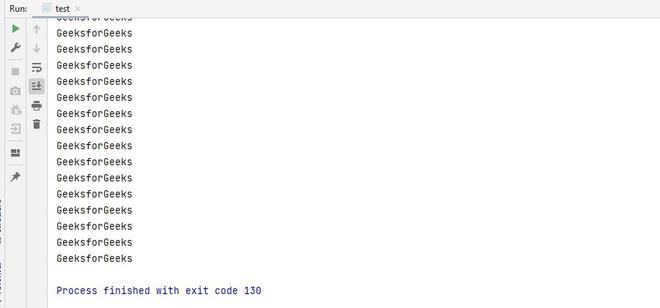In the above code, the program should ideally stop if Return Key/Enter is pressed, but in some machines, it may happen that the variable running is cached, and we are not able to change its value using the shutDown() method. In such a case, the program will execute infinite times and cannot be exited properly. To avoid caching and make it Thread-safe, we can use volatile keywords while declaring the running variable.

## Java

 `import` `java.io.*;``import` `java.util.*;`` ` `class` `Geeks ``extends` `Thread{``    ``volatile` `boolean` `running=``true``;``    ``@Override``    ``public` `void` `run(){``        ``while``(running){``            ``System.out.println(``"GeeksforGeeks"``);``        ``}``    ``}``    ``public` `void` `shutDown(){``        ``running=``false``;``    ``}``}`` ` `class` `GFG{``    ``public` `static` `void` `main(String[] args) {``        ``Geeks obj = ``new` `Geeks();``        ``obj.start();``        ``Scanner input = ``new` `Scanner(System.in);``        ``input.nextLine();``        ``obj.shutDown();``    ``}``}`

Output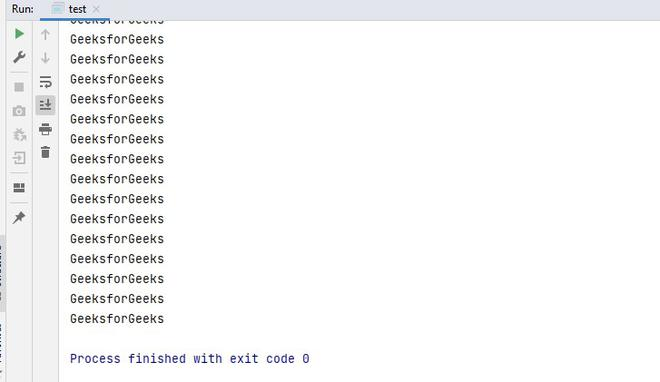In the above code, after using the volatile keyword, we can stop the infinite loop using the Return key, and the program exited properly with exit code 0.

### 6. transient

This needs prior knowledge of serialization in Java. You can refer to the following article for that:- serialization in java.

The transient keyword may be applied to member variables of a class to indicate that the member variable should not be serialized when the containing class instance is serialized. Serialization is the ​process of converting an object into a byte stream. When we do not want to serialize the value of a variable, then we declare it as transient. To make it more transparent, let’s take an example of an application where we need to accept UserID and Password. At that moment, we need to declare some variable to take the input and store the data, but as the data is susceptible, so we do not want to keep it stored after the job is done. To achieve this, we can use the transient keyword for variable declaration. That particular variable will not participate in the serialization process, and when we deserialize that, we will receive the default value of the variable. Let’s see a sample code for the same –

## Java

 `import` `java.io.*;`` ` `class` `transient_gfg ``implements` `Serializable {``    ``// normal variable``    ``int` `a = ``10``; ``     ` `    ``// Transient variables``    ``transient` `String UserID=``"admin"``; ``    ``transient` `String Password=``"tiger123"``;`` ` `}``class` `GFG{``    ``public` `static` `void` `main(String[] args) ``throws` `IOException, ClassNotFoundException {``        ``transient_gfg obj=``new` `transient_gfg();``       ` `        ``// printing the value of transient``        ``// variable before serialization process``        ``System.out.println(``"UserID :"``+obj.UserID);``        ``System.out.println(``"Password: "``+obj.Password);``        ``System.out.println(``"a = "``+obj.a);`` ` `        ``// serialization``        ``FileOutputStream fos = ``new` `FileOutputStream(``"abc.txt"``);``        ``ObjectOutputStream oos = ``new` `ObjectOutputStream(fos);``        ``oos.writeObject(obj);``       ` `        ``// de-serialization``        ``FileInputStream fis = ``new` `FileInputStream(``"abc.txt"``);``        ``ObjectInputStream ois = ``new` `ObjectInputStream(fis);``        ``transient_gfg output = (transient_gfg)ois.readObject();``       ` `        ``// printing the value of transient ``        ``// variable after de-serialization process``        ``System.out.println(``"UserID :"``+output.UserID);``        ``System.out.println(``"Password: "``+output.Password);``        ``System.out.println(``"a = "``+obj.a);`` ` `    ``}``}`

Output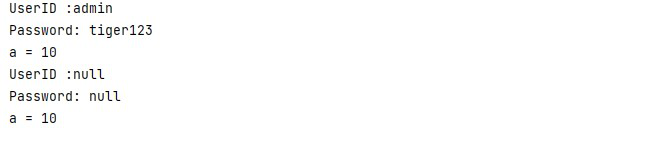As you see from the output, after serialization, the values of UserID and Password are no longer present. However, the value of ‘a’, which is a normal variable, is still present.

### 7. native

The native keyword may be applied to a method to indicate that the method is implemented in a language other than Java. Using this java application can call code written in C, C++, or assembler language. A shared code library or DLL is required in this case. Let’s see an example first –

## Java

 `import` `java.io.*;`` ` `class` `GFG``{``    ``// native method``    ``public` `native` `void` `printMethod (); ``    ``static``    ``{``        ``// The name of DLL file``        ``System.loadLibrary (``"LibraryName"``);  ``    ``}``    ``public` `static` `void` `main (String[] args)``    ``{``        ``GFG obj = ``new` `GFG ();``        ``obj.printMethod ();``    ``}``}`

Output: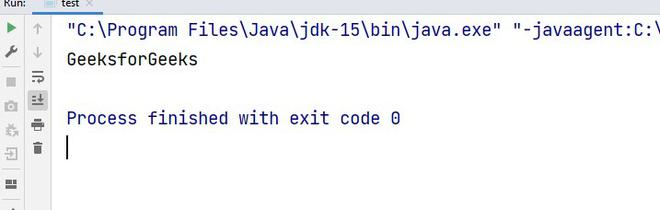In the above code, we have a native method. The method is defined in any other language, loaded by a java application using the shared DLL file. Implementation of the DLL file is out of scope for this article, so if you want to know more about it, you can refer to this article – Multi-Language Programming – Java Process Class, JNI, and IO.

My Personal Notes arrow_drop_up